# Solution assignment 01 Partial differentiation

### Assignment 1

Calculate the first, second and mixed partial derivatives of the following function.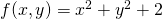### Solution

This is a relatively simple function. We get: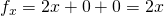(consider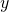as a constant)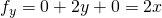(consideras a constant)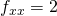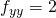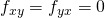Indeed, we notice that the mixed derivatives are equal.